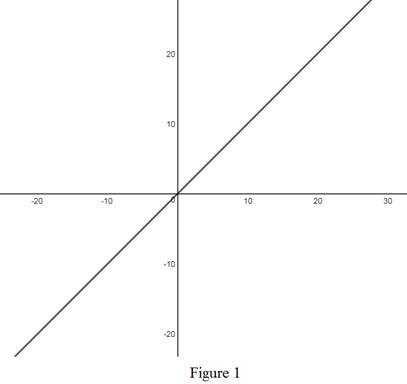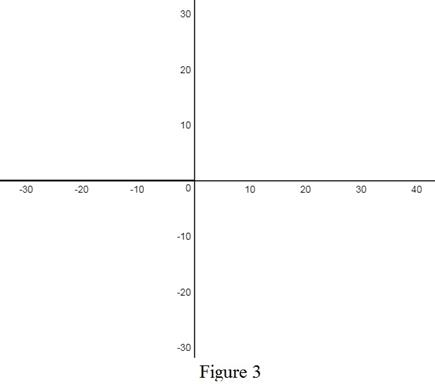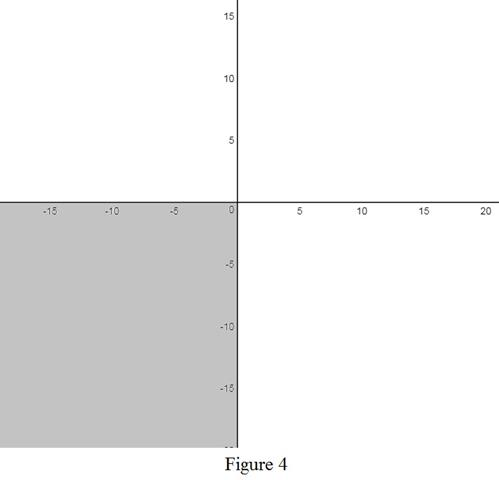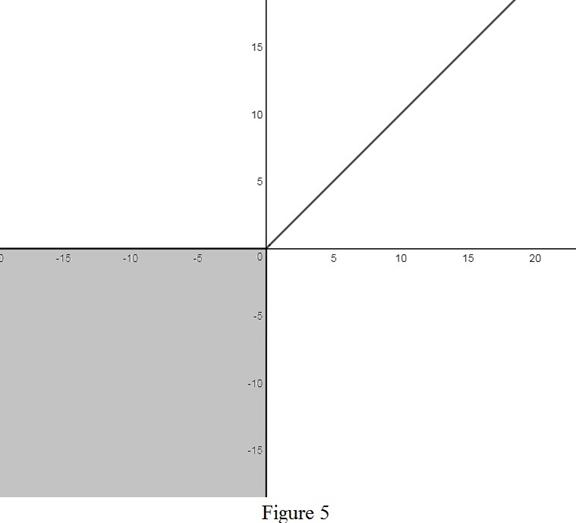# The equation x + | x | = y + | y | .### Single Variable Calculus: Concepts...

4th Edition
James Stewart
Publisher: Cengage Learning
ISBN: 9781337687805### Single Variable Calculus: Concepts...

4th Edition
James Stewart
Publisher: Cengage Learning
ISBN: 9781337687805

#### Solutions

Chapter 1, Problem 7P
To determine

## To sketch: The equation x+|x|=y+|y|.

Expert Solution

### Explanation of Solution

Definition:

The absolute value is defined as, |x|={xif x0xif x<0.

Calculation:

Consider the equation x+|x|=y+|y|.

Take, x+|x|={2xifx00ifx<0

Similarly, y+|y|={2yify00ify<0

Case (i):x0 and y0

The corresponding equation for this case is,

2x=2yx=y

To sketch:

Use the online graphing calculator and draw the graph as shown below in Figure 1.From Figure 1, observe that the graph lies in first quadrant as x and y values are positive.

Case (ii):x0 and y<0

The corresponding equation for this case is,

2x=0x=0

To sketch:

Use the online graphing calculator and draw the graph as shown below in Figure 2.From Figure 2, observe that the graph is negative y axis.

Case (iii):x<0 and y0 The corresponding equation for this case is,

0=2yy=0

To sketch: Use the online graphing calculator and draw the graph as shown below in Figure 3From Figure 3, observe that the graph is the negative x axis.

Case (iv): x<0 and y<0 This gives the entire third quadrant.From the above four cases, the graph of x+|x|=y+|y| is shown below in Figure 4.From Figure 5, it is observed that the graph contains the entire third quadrant.

### Have a homework question?

Subscribe to bartleby learn! Ask subject matter experts 30 homework questions each month. Plus, you’ll have access to millions of step-by-step textbook answers!